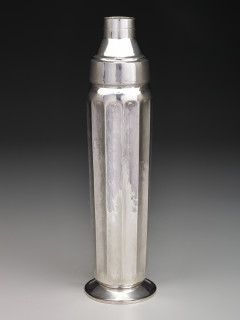# Dallas Museum of Art presents Runo Lagomarsino: "Concentrations 61"Photo courtesy of Dallas Museum of Art

"Concentrations 61" is the first solo museum exhibition for Runo Lagomarsino, a conceptual artist of Italian and Argentine descent who lives and works in Malmö, Sweden, and São Paulo, Brazil. His work has been exhibited in group shows at the Guggenheim, the Los Angeles County Museum of Art, MCA Chicago, PROSPECT, and the Venice Biennale, among others.

Transforming everyday objects and phrases into historically referential works of art, Lagomarsino considers the unstable nature of national identities. Through these deceptively simple transformations, the artist points to the histories and narratives imparted by our transnational identities and the volatile relationship between power and geography.

"Concentrations 61" spans two galleries and features new commissions and previous works that have been specifically reformatted for the DMA.

### Event Details

#### When

4.14.19 | 11:00 am
4.13.19 | 11:00 am
4.12.19 | 11:00 am
+ see all dates and times
4.11.19 | 11:00 am
4.10.19 | 11:00 am
4.9.19 | 11:00 am
4.7.19 | 11:00 am
4.6.19 | 11:00 am
4.5.19 | 11:00 am
4.4.19 | 11:00 am
4.3.19 | 11:00 am
4.2.19 | 11:00 am
3.31.19 | 11:00 am
3.30.19 | 11:00 am
3.29.19 | 11:00 am
3.28.19 | 11:00 am
3.27.19 | 11:00 am
3.26.19 | 11:00 am
3.24.19 | 11:00 am
3.23.19 | 11:00 am
3.22.19 | 11:00 am
3.21.19 | 11:00 am
3.20.19 | 11:00 am
3.19.19 | 11:00 am
3.17.19 | 11:00 am
3.16.19 | 11:00 am
3.15.19 | 11:00 am
3.14.19 | 11:00 am
3.13.19 | 11:00 am
3.12.19 | 11:00 am
3.10.19 | 11:00 am
3.9.19 | 11:00 am
3.8.19 | 11:00 am
3.7.19 | 11:00 am
3.6.19 | 11:00 am
3.5.19 | 11:00 am
3.3.19 | 11:00 am
3.2.19 | 11:00 am
3.1.19 | 11:00 am
2.28.19 | 11:00 am
2.27.19 | 11:00 am
2.26.19 | 11:00 am
2.24.19 | 11:00 am
2.23.19 | 11:00 am
2.22.19 | 11:00 am
2.21.19 | 11:00 am
2.20.19 | 11:00 am
2.19.19 | 11:00 am
2.17.19 | 11:00 am
2.16.19 | 11:00 am
2.15.19 | 11:00 am
2.14.19 | 11:00 am
2.13.19 | 11:00 am
2.12.19 | 11:00 am
2.10.19 | 11:00 am
2.9.19 | 11:00 am
2.8.19 | 11:00 am
2.7.19 | 11:00 am
2.6.19 | 11:00 am
2.5.19 | 11:00 am
2.3.19 | 11:00 am
2.2.19 | 11:00 am
2.1.19 | 11:00 am
1.31.19 | 11:00 am
1.30.19 | 11:00 am
1.29.19 | 11:00 am
1.27.19 | 11:00 am
1.26.19 | 11:00 am
1.25.19 | 11:00 am
1.24.19 | 11:00 am
1.23.19 | 11:00 am
1.22.19 | 11:00 am
1.20.19 | 11:00 am
1.19.19 | 11:00 am
1.18.19 | 11:00 am
1.17.19 | 11:00 am
1.16.19 | 11:00 am
1.15.19 | 11:00 am
1.13.19 | 11:00 am
1.12.19 | 11:00 am
1.11.19 | 11:00 am
1.10.19 | 11:00 am
1.9.19 | 11:00 am
1.8.19 | 11:00 am
1.6.19 | 11:00 am
1.5.19 | 11:00 am
1.4.19 | 11:00 am
1.3.19 | 11:00 am
1.2.19 | 11:00 am
12.30.18 | 11:00 am
12.29.18 | 11:00 am
12.28.18 | 11:00 am
12.27.18 | 11:00 am
12.26.18 | 11:00 am
12.23.18 | 11:00 am
12.22.18 | 11:00 am
12.21.18 | 11:00 am
12.20.18 | 11:00 am
12.19.18 | 11:00 am
12.18.18 | 11:00 am
12.16.18 | 11:00 am
12.15.18 | 11:00 am
12.14.18 | 11:00 am
12.13.18 | 11:00 am
12.12.18 | 11:00 am
12.11.18 | 11:00 am
12.9.18 | 11:00 am
12.8.18 | 11:00 am
12.7.18 | 11:00 am
12.6.18 | 11:00 am
12.5.18 | 11:00 am
12.4.18 | 11:00 am
12.2.18 | 11:00 am
12.1.18 | 11:00 am
11.30.18 | 11:00 am
11.29.18 | 11:00 am
11.28.18 | 11:00 am
11.27.18 | 11:00 am
11.25.18 | 11:00 am
11.24.18 | 11:00 am
11.23.18 | 11:00 am
11.21.18 | 11:00 am
11.20.18 | 11:00 am
11.18.18 | 11:00 am
11.17.18 | 11:00 am
11.16.18 | 11:00 am
11.15.18 | 11:00 am
11.14.18 | 11:00 am
11.13.18 | 11:00 am
11.11.18 | 11:00 am
11.10.18 | 11:00 am
11.9.18 | 11:00 am
11.8.18 | 11:00 am
11.7.18 | 11:00 am
11.6.18 | 11:00 am
11.4.18 | 11:00 am
11.3.18 | 11:00 am
11.2.18 | 11:00 am
11.1.18 | 11:00 am
10.31.18 | 11:00 am
10.30.18 | 11:00 am
10.28.18 | 11:00 am
10.27.18 | 11:00 am
10.26.18 | 11:00 am
10.25.18 | 11:00 am
10.24.18 | 11:00 am
10.23.18 | 11:00 am
10.21.18 | 11:00 am
10.20.18 | 11:00 am
10.19.18 | 11:00 am
10.18.18 | 11:00 am
10.17.18 | 11:00 am
10.16.18 | 11:00 am
10.14.18 | 11:00 am
10.13.18 | 11:00 am
10.12.18 | 11:00 am
10.11.18 | 11:00 am
10.10.18 | 11:00 am
10.9.18 | 11:00 am
10.7.18 | 11:00 am
10.6.18 | 11:00 am
10.5.18 | 11:00 am
10.4.18 | 11:00 am
10.3.18 | 11:00 am
10.2.18 | 11:00 am
9.30.18 | 11:00 am

#### Where

Dallas Museum of Art
1717 N. Harwood St.
Dallas, TX 75201